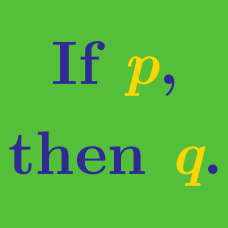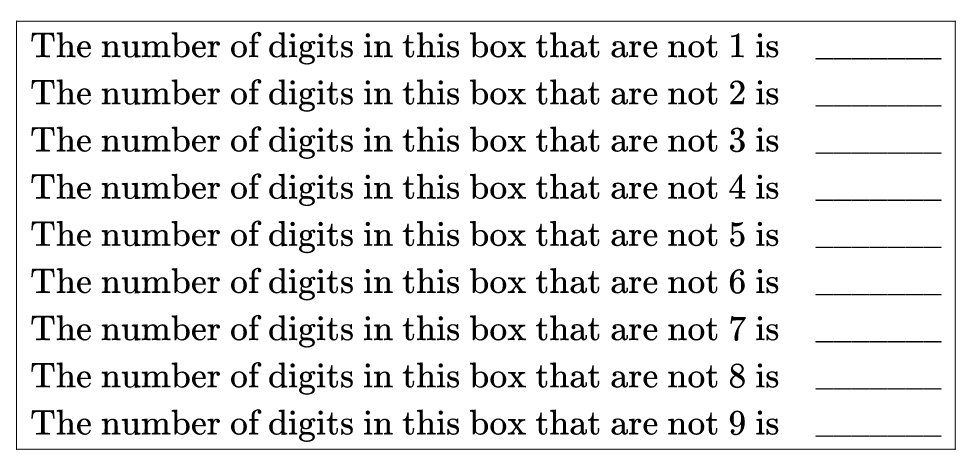Logic

# Logic Warmups: Level 5 Challenges

There are 100 islands, each of a distinct size. 100 perfectly logical villagers live on each island, and the numbers of villagers with blue eyes on each of the islands are the distinct numbers $0,1,\ldots,99$, with a uniform distribution.

On one of the islands, all the villagers know that everyone has either blue or brown eyes, but it is forbidden for a villager to know his/her own eye color. If a villager believes they know their own eye color on a given day, they must leave the village in exile that night.

One day a fairy visits this island and announces that at least 50 villagers have blue eyes. Unfortunately, the fairy chooses completely randomly whether to lie or to the truth, but each villager believes the fairy unless they observe a contradiction. The villagers do not discuss the fairy's statement.

What is the probability that a given random villager from the smallest island will eventually leave the island in exile but be incorrect about their eye color?

If the probability is $\frac{p}{q}$, where $p,q$ are positive, coprime integers, give your answer as $p+q$.From top to bottom, concatenate the nine numbers that is in the blank spaces as your answer.

You are given a balance scale without measurements, which can determine the order of increasing distinct weights in just one weighing. However, if we have 3 objects, then we will need 3 weighings to determine the relative order of their weights.

Now suppose you have been asked to rank 9 given objects in order of increasing weights, what is the minimum number of weighing needed to guarantee that we can perform this task?

Arrange the numbers $1 - 32$, inclusive, in a circle such that the sum of any two adjacent numbers in the circular chain is a perfect square.

When you crack the Mystic Circle, you will obtain $32$ Square numbers which are the sums of adjacent numbers.

If the number of times the squares $4, 9, 16, 25, 36, 49$ appear is given by $A. B, C, D, E, F$ respectively, find $1A + 2B + 3C + 4D + 5E + 6F$.

$\large \overline{A_1 A_2 A_3 \ldots A_{16}} \times 150\% = \overline{A_2 A_3 \ldots A_{15}A_{16} A_1}$

How many distinct 16-digit positive integer(s) satisfy the condition that if I move its first digit to the last digit, the resultant number increases by 50%?

×

Problem Loading...

Note Loading...

Set Loading...